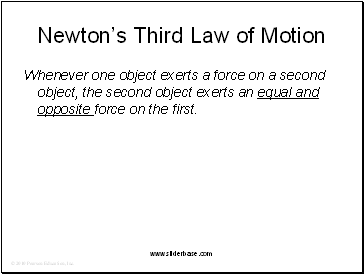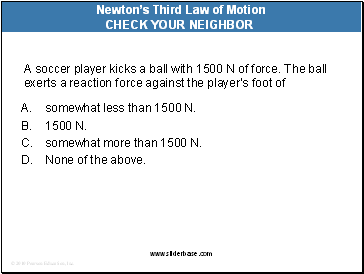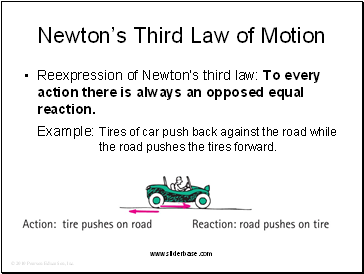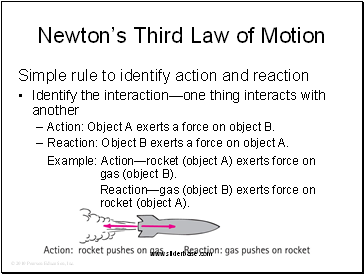# Newton’s third law of motionPage 1

#### WATCH ALL SLIDES

Slide 1Forces and Interactions

Newton’s Third Law of Motion

Summary of Newton’s Laws

Vectors

Slide 2## Forces and Interactions

Interaction

is between one thing and another.

requires a pair of forces acting on two objects.

Example: interaction of hand and wall pushing on each other

Force pair—you push on wall; wall pushes on you.

Slide 3## Newton’s Third Law of Motion

Whenever one object exerts a force on a second object, the second object exerts an equal and opposite force on the first.

Slide 4A soccer player kicks a ball with 1500 N of force. The ball exerts a reaction force against the player’s foot of

A. somewhat less than 1500 N.

1500 N.

somewhat more than 1500 N.

None of the above.

Newton’s Third Law of Motion

Slide 5A soccer player kicks a ball with 1500 N of force. The ball exerts a reaction force against the player’s foot of

A. somewhat less than 1500 N.

1500 N.

somewhat more than 1500 N.

None of the above.

Newton’s Third Law of Motion

Slide 6Newton’s Third Law of Motion

Action and reaction forces

one force is called the action force; the other force is called the reaction force.

are co-pairs of a single interaction.

neither force exists without the other.

are equal in strength and opposite in direction.

always act on different objects.

Slide 7Newton’s Third Law of Motion

Reexpression of Newton’s third law: To every action there is always an opposed equal reaction.

Example: Tires of car push back against the road while the road pushes the tires forward.

Slide 8Newton’s Third Law of Motion

Simple rule to identify action and reaction

Identify the interaction—one thing interacts with another

Action: Object A exerts a force on object B.

Reaction: Object B exerts a force on object A.

Example: Action—rocket (object A) exerts force on gas (object B).

Reaction—gas (object B) exerts force on rocket (object A).

Slide 9When you step off a curb, Earth pulls you downward. The reaction to this force is

Go to page:
1  2  3  4  5  6